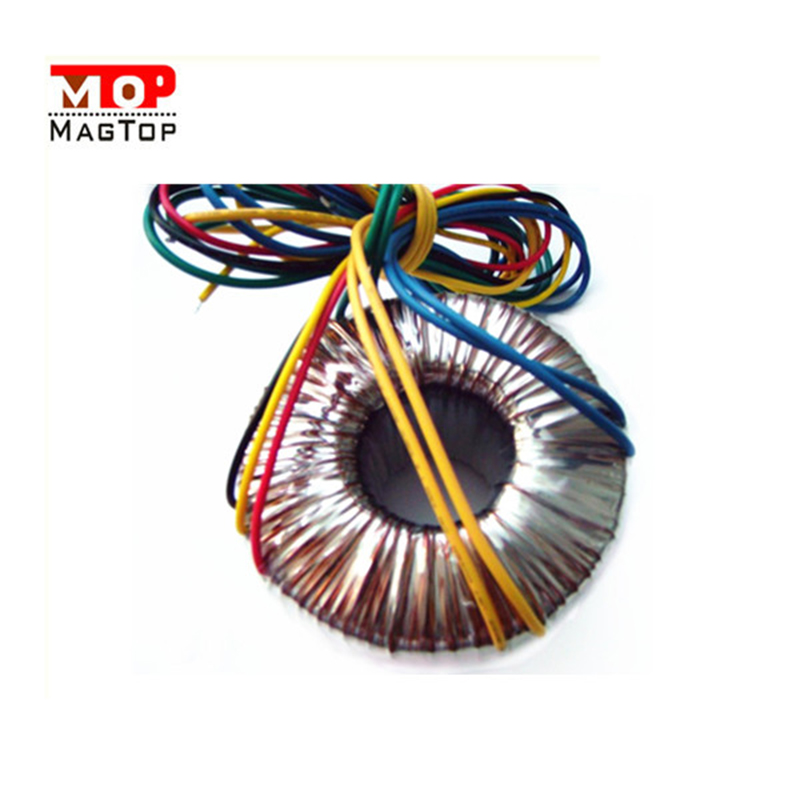# Characteristics of power transformers

##### author: KZ
21/09/20221. Operating frequency The core loss of the transformer has a great relationship with the frequency, so it should be designed and used according to the frequency of use. This frequency is called the operating frequency.
2. Rated power Under the specified frequency and voltage, the transformer can work for a long time without exceeding the output power of the specified temperature rise.
3. Rated voltage refers to the voltage allowed to be applied to the coil of the transformer, which shall not be greater than the specified value during operation.
4. Voltage ratio refers to the ratio of the primary voltage to the secondary voltage of the transformer, and there is a difference between the no-load voltage ratio and the load voltage ratio.
5. No-load current When the secondary of the transformer is open, there is still a certain current in the primary, and this part of the current is called the no-load current. The no-load current consists of the magnetizing current (which produces the magnetic flux) and the iron loss current (caused by core losses). For a 50Hz power transformer, the no-load current is essentially equal to the magnetizing current.
6. No-load loss refers to the power loss measured at the primary when the secondary of the transformer is open. The main loss is the iron core loss, followed by the loss (copper loss) generated by the no-load current on the copper resistance of the primary coil, and this part of the loss is very small.
7. Efficiency refers to the percentage of the ratio of the secondary power P2 to the primary power P1. Generally, the higher the rated power of the transformer, the higher the efficiency.
8. Insulation resistance Indicates the insulation performance between the coils of the transformer and between the coils and the iron core. The level of insulation resistance is related to the performance, temperature and humidity of the insulating material used.